• Unity内建矩阵类型：M：世界矩阵V：摄像机矩阵P：投影矩阵T ：矩阵的转置IT : 转置的的逆_Object2World: 模型到世界矩阵_World2Object:世界到模型矩阵MVP 矩阵变换：Shader DemoMVP 转置变换：C# demoMatrix4x4 mvp ...
Unity内建矩阵类型：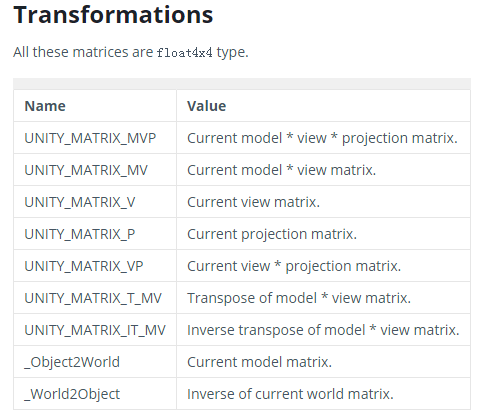M：世界矩阵V：摄像机矩阵P：投影矩阵T ：矩阵的转置IT : 转置的的逆_Object2World: 模型到世界矩阵_World2Object:世界到模型矩阵MVP 矩阵变换：Shader Demo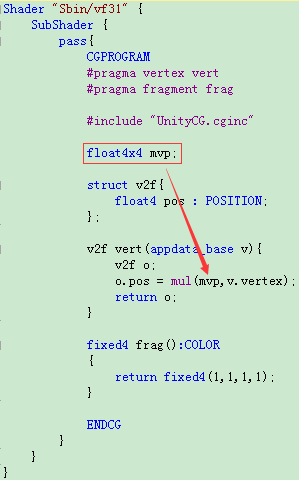MVP 转置变换：C# demo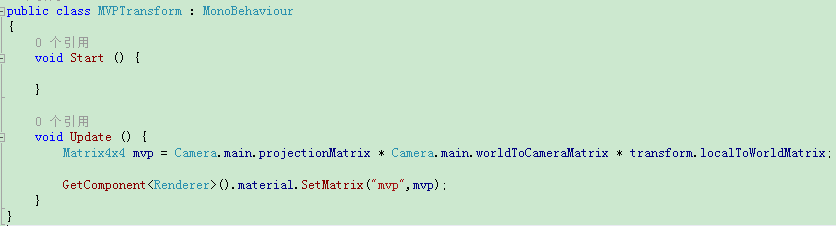Matrix4x4 mvp = Camera.main .projectionMatrix * Camera.main .worldToCameraMatrix * transform .localToWorldMatrix;矩阵相乘是有顺序的，如果没有真正显示，就逆着相乘看下效果。最后对shader 的mvp 属性赋值mvp 矩阵，而不使用unity内建的UNITY_MATRIX_MVP矩阵
展开全文• ViewMatrix用于直接将World坐标系下的坐标转换到Camera坐标系下。已知相机的坐标系，还有相机在世界空间下的坐标.就可以求出ViewMatrix，下面进行具体推导。令UVN为相机坐标系下的三个基，，对于一个相机来说，它在...
矩阵推导ViewMatrix用于直接将World坐标系下的坐标转换到Camera坐标系下。已知相机的坐标系，还有相机在世界空间下的坐标.就可以求出ViewMatrix，下面进行具体推导。令UVN为相机坐标系下的三个基，，对于一个相机来说，它在开始的时候和世界坐标系是重合的，用户控制相机在世界空间中移动之后，相机的状态可以用两个属性来描述——朝向和位置。也就是说，有了这两个属性，一个相机模型在世界中的状态就确定了。而这两个属性，我们用变换的理论来描述，就是旋转和平移。可以想象，对于世界中的任何一个相机状态，我们都可以把它看成是：相机先围绕自身基原点旋转一定的角度，然后平移到世界空间的某个地方。下图展示了这个过程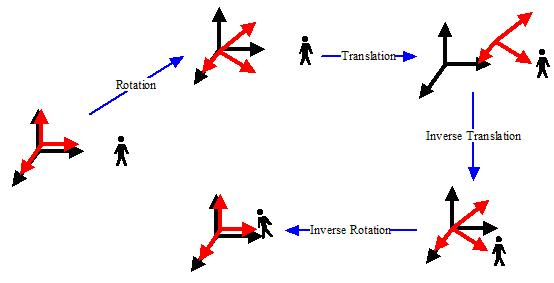图一 . 相机的变换与逆变换	图中，红色是相机的基，而黑色是世界的基，也就是参考系。小人是世界中的一个物体。相机在移动之前，两个基是重合的。当相机在屏幕中定位时，它首先会进行朝向的确定——旋转，然后进行位置的确定——平移。图中的Rotation和Translation两步就是相机定位时所发生的变换。可以看到相机相对于小人的运动。而当进行相机变换的时候，小人应该从世界基变换到相机的基里面。这样，他应该进行一个相机定位的逆定位，先逆平移小人和相机，然后再逆旋转小人和相机，最后相机归位，小人随相机变到了相机空间。这是由Inverse Translation和Inverse Rotation两个步骤完成的，这两个步骤就是相机变换。现在我们推导这个变换。我们把关系写出来，相机本身的变换C包括两个元素C = TR其中T是平移变换，R是旋转变换。而相机变换是相机本身变换的逆变换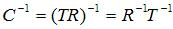这个C^-1就是我们要求出的相机变换。其中T^-1很容易求出，即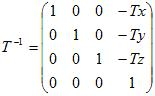R^(-1)比较难求出，这里用到了正交基一些知识，可以参考下碰撞检测之Ray-Cylinder检测前面关于正交基的部分。当相机变换进行完Inverse Translation这一步之后，相机的原点和世界原点就重合了，也就是处理完了关于平移的变换。接下来我们要做的是逆旋转，而其实逆旋转的目的，就是要得到目前世界坐标中经过逆平移的小人在相机坐标系中的坐标。由坐标转换公式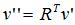对于世界坐标中的已经经过逆平移的坐标v’，它在相机坐标系R中的坐标是v’’，而相机坐标系就是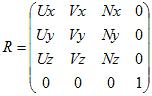则相机变换的完整公式就是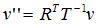其中v是小人在世界空间中的坐标，v’’是小人在相机空间中的坐标。则相机变换矩阵就是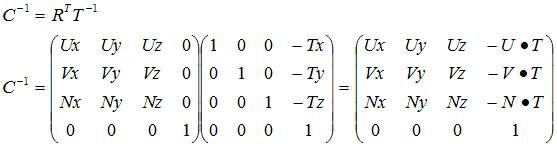常见的求ViewMatrix的情况有三种，一种是用LookAt函数，第二种是类似FPS游戏中通过pitch和yaw来算，还有一种是类似轨迹球的算法。最后的方法都是转化为求出相机的坐标系的基。Look At Camera这里参考是左手坐标系，而且在相机空间，相机的forward是Z轴的负方向，和Unity还有OpenGL一致。图二 . 相机的左手坐标系如下图，这种求法需要知道摄像机的位置 eye，一个up向量（global）,还有摄像机的观察点at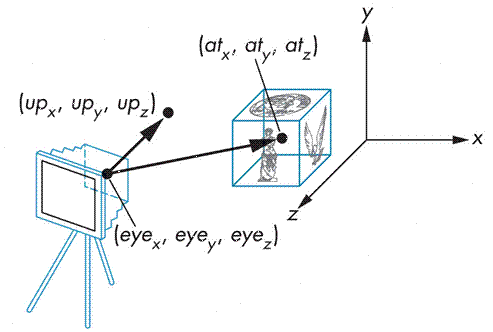图三 . LookAt函数模型Matrix4x4 LookAt(const Vector3& eye, const Vector3& target, const Vector3& up)
{
Vector3 z((eye - target).normalized());
Vector3 x((Vector3::Cross(z, up).normalized()));
Vector3 y(Vector3::Cross(x, z));

Matrix4x4 result;

result = x.x;
result = x.y;
result = x.z;
result = -Vector3::Dot(x, eye);

result = y.x;
result = y.y;
result = y.z;
result = -Vector3::Dot(y, eye);

result = z.x;
result = z.y;
result = z.z;
result = -Vector3::Dot(z, eye);

result = result = result = 0.0f;
result = 1.0f;
return result;
}算例//C++
qDebug() << Transform::LookAt(Vector3(1, 2, 3), Vector3(0, 10, 0), Vector3::up);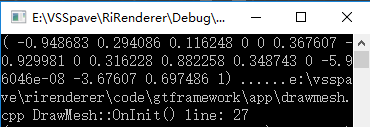图四 . LookAt函数计算结果//Unity
transform.position = new Vector3(1, 2, 3);
transform.LookAt(new Vector3(0, 10, 0), Vector3.up);
Debug.Log(Camera.main.worldToCameraMatrix);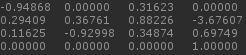图五 . Unity中的计算结果（貌似Unity中的Transform.LookAt函数是直接修改的Camera的Rotation？）FPS Camera通过之前的结论假设原始的坐标系为x-(1,0,0), y-(0,1,0), z(0,0,1),这个坐标系经过一个旋转矩阵Matrix旋转之后，新的坐标系就是这个旋转矩阵的转置三个列向量。其实这里要求的只是旋转矩阵的转置。注意相机的Z方向并不是相机正对的方向，而是相机的背面。Matrix4x4 Transform::FPSView(const Vector3& eye, Quaternion rotation)
{
Matrix4x4 rotMatrix = rotation.GetRotMatrix().transpose();
Vector3 x(rotMatrix, rotMatrix, rotMatrix);
Vector3 y(rotMatrix, rotMatrix, rotMatrix);
Vector3 z(-rotMatrix, -rotMatrix, -rotMatrix);

Matrix4x4 result;

result = x.x;
result = x.y;
result = x.z;
result = -Vector3::Dot(x, eye);

result = y.x;
result = y.y;
result = y.z;
result = -Vector3::Dot(y, eye);

result = z.x;
result = z.y;
result = z.z;
result = -Vector3::Dot(z, eye);

result = result = result = 0.0f;
result = 1.0f;
return result;
}算例//C++
qDebug() << Transform::FPSView(Vector3(1,2,3), Quaternion::Euler(30, 45, 60));结果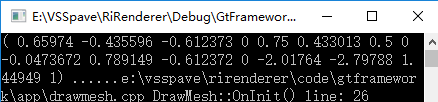图六 . FPV函数计算结果//unity
transform.position = new Vector3(1, 2, 3);
transform.rotation = Quaternion.Euler(30, 45, 60);
Debug.Log(Camera.main.worldToCameraMatrix);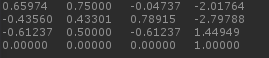图七 . Unity中运行结果参考Understanding the View Matrix - http://www.3dgep.com/understanding-the-view-matrix/Tutorial 3 : Matrices - http://www.opengl-tutorial.org/beginners-tutorials/tutorial-3-matrices/OpenGL Transformation - http://www.songho.ca/opengl/gl_transform.html推导相机变换矩阵 - http://blog.csdn.net/popy007/article/details/5120158链接详解MVP矩阵之齐次坐标和ModelMatrix详解MVP矩阵之齐次坐标和ViewMatrix详解MVP矩阵之齐次坐标和ProjectionMatrix
展开全文unity3d 算法
• 内置矩阵 支持的矩阵（float4x4）： ...UNITY_MATRIX_MVP 当前模型视图投影矩阵 UNITY_MATRIX_MV 当前模型视图矩阵 UNITY_MATRIX_V 当前视图矩阵UNITY_MATRIX_P 目前的投影矩阵 UNITY_MATRIX_VP 当前

内置矩阵 支持的矩阵（float4x4）：

UNITY_MATRIX_MVP        当前模型视图投影矩阵
UNITY_MATRIX_MV           当前模型视图矩阵
UNITY_MATRIX_V              当前视图矩阵。
UNITY_MATRIX_P              目前的投影矩阵
UNITY_MATRIX_VP            当前视图*投影矩阵
UNITY_MATRIX_T_MV       移调模型视图矩阵
UNITY_MATRIX_IT_MV      模型视图矩阵的逆转
UNITY_MATRIX_TEXTURE0   UNITY_MATRIX_TEXTURE3          纹理变换矩阵

内置载体
Vectors (float4) supported:

向量（仅float4）支持：

UNITY_LIGHTMODEL_AMBIENT        当前环境的颜色。

展开全文• 含义 UNITY_MATRIX_MVP 当前模型视图投影矩阵 UNITY_MATRIX_MV 当前模型视图矩阵 UNITY_MATRIX_V 当前视图矩阵 UNITY_MATRIX_P 目前的投影矩阵 UNITY_MATRIX_VP 当前视图*投影矩阵 UNITY_MATRIX_T_MV 移...
内置矩阵变量
含义
UNITY_MATRIX_MVP
当前模型视图投影矩阵
UNITY_MATRIX_MV
当前模型视图矩阵
UNITY_MATRIX_V
当前视图矩阵
UNITY_MATRIX_P
目前的投影矩阵
UNITY_MATRIX_VP
当前视图*投影矩阵
UNITY_MATRIX_T_MV
移调模型视图矩阵
UNITY_MATRIX_IT_MV
模型视图矩阵的逆转

展开全文unity
• 简介透视投影是3D固定流水线的重要组成部分，是将相机空间中的点从视锥体(frustum)变换到规则观察体(Canonical View Volume)中，待裁剪完毕后进行透视除法的行为。在算法中它是通过透视矩阵乘法和透视除法两步完成的...
简介透视投影是3D固定流水线的重要组成部分，是将相机空间中的点从视锥体(frustum)变换到规则观察体(Canonical View Volume)中，待裁剪完毕后进行透视除法的行为。在算法中它是通过透视矩阵乘法和透视除法两步完成的。 具体的理论推导过程可以参考下面的几个Link，深入探索透视投影变换深入探索透视投影变换(续)OpenGL TransformationOpenGL ProjectionMatrix推导正交投影变换 代码实现 首先是Perspective的Camera，用Unity的Camera来作为参照。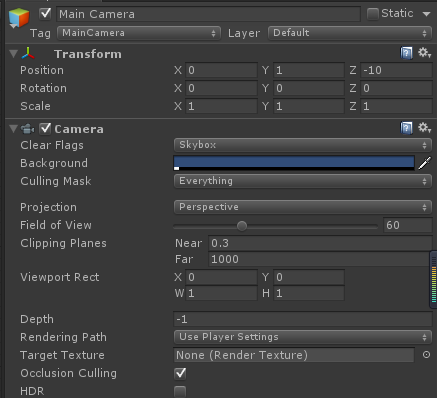(0,0,0)点放一个quad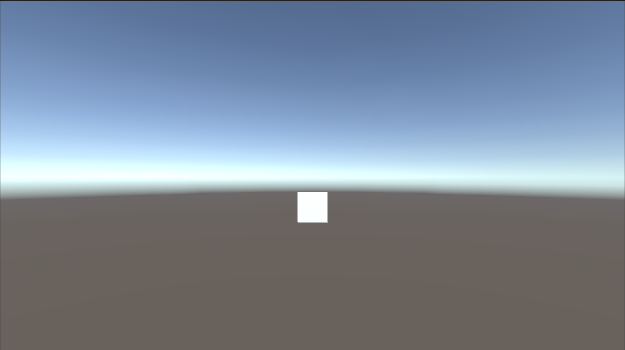加一个脚本来测试一下 Debug.Log("ProjectionMatrix\n" + GetComponent<Camera>().projectionMatrix);
Debug.Log("ViewMatrix\n" + GetComponent<Camera>().worldToCameraMatrix);
Debug.Log("ModeMatrix\n" + mesh.transform.localToWorldMatrix);     打印出相机的矩阵，结果如下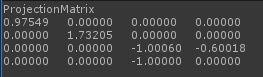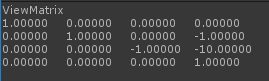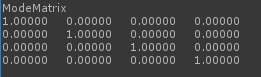打印quad各个顶点的位置。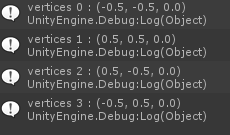经过计算P * V *M * Vector4(pos, 1) ,得到结果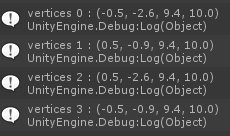C++ 代码camera = new Camera(Vector3(0, 1, -10), 60,16.0f / 9, 0.3f, 1000);
Matrix4x4 model = Matrix4x4::identity;

qDebug() <<"ProjectionMatri: " << camera->GetProjectionMatrix();
qDebug() << "ViewMatrix: " << camera->GetViewMatrix();
qDebug() << "ModelMatrix: " << model;

Vector3 vertice0(-0.5f, -0.5F, 0);
Vector3 vertice1(0.5f, 0.5F, 0);
Vector3 vertice2(0.5f, -0.5F, 0);
Vector3 vertice3(-0.5f, 0.5F, 0);

Matrix4x4 mvp = camera->GetProjectionMatrix() * camera->GetViewMatrix() * model;
//Vector3 tmp = mvp * vertice0;
qDebug() << "vertice0" << mvp * Vector4(vertice0.x, vertice0.y, vertice0.z, 1);
qDebug() << "vertice1" << mvp * Vector4(vertice1.x, vertice1.y, vertice1.z, 1);
qDebug() << "vertice2" << mvp * Vector4(vertice2.x, vertice2.y, vertice2.z, 1);
qDebug() << "vertice3" << mvp * Vector4(vertice3.x, vertice3.y, vertice3.z, 1);
结果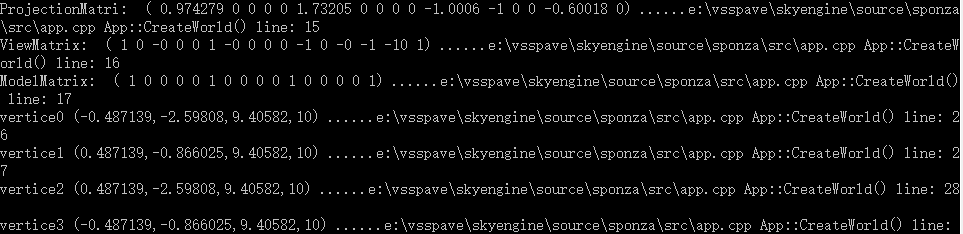其中Perspective函数如下Matrix4x4 Transform::Perspective(float fovy, float aspect, float zNear, float zFar)
{

float tanHalfFovy = tan(Mathf::Deg2Rad * fovy / 2);

Matrix4x4 result(0.0f);
result[0 * 4 + 0] = 1.0f / (aspect * tanHalfFovy);
result[1 * 4 + 1] = 1.0f / (tanHalfFovy);
result[2 * 4 + 3] = -1.0f;

result[2 * 4 + 2] = -(zFar + zNear) / (zFar - zNear);
result[3 * 4 + 2] = -(2.0f * zFar * zNear) / (zFar - zNear);
return result;
}
同样功能的还有Frustum函数Matrix4x4 Transform::Frustum(float l, float r, float b, float t, float zNear, float zFar)
{
Matrix4x4 result(0.0f);

result = 2 * zNear / (r - l);
result = (r + l) / (r - l);
result = 2 * zNear / (t - b);
result = (t + b) / (t - b);
result = -(zFar + zNear) / (zFar - zNear);
result = -(2 * zFar * zNear) / (zFar - zNear);
result = -1;
result = 0;
return result;
} Opengl的流水线如下图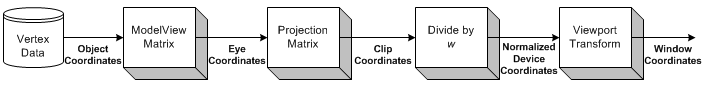乘完了MVP矩阵，得到的是Clip Coordinates。坐标除以w之后（称为透视除法），得到了NDC坐标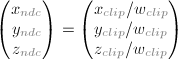最后通过线性变换，得到最终的屏幕空间坐标。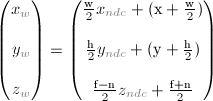Opengl里面设置view port通常是这样glViewport(x, y, w, h);
glDepthRange(n, f);对应关系如下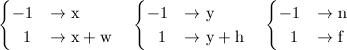还是继续上面的例子进行计算 同除以w之后，得到NDC坐标
Vector3 ndcPos0 = Vector3(clipPos0.x / clipPos0.w, clipPos0.y / clipPos0.w, clipPos0.z / clipPos0.w);
Vector3 ndcPos1 = Vector3(clipPos1.x / clipPos1.w, clipPos1.y / clipPos1.w, clipPos1.z / clipPos1.w);
Vector3 ndcPos2 = Vector3(clipPos2.x / clipPos2.w, clipPos2.y / clipPos2.w, clipPos2.z / clipPos2.w);
Vector3 ndcPos3 = Vector3(clipPos3.x / clipPos3.w, clipPos3.y / clipPos3.w, clipPos3.z / clipPos3.w);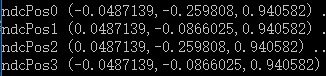在一个640* 360 的窗口中，    int x = 0, y = 0, w = 640, h = 360, n = 0.3, f =1000;
Vector3 screenPos0(w * 0.5f * ndcPos0.x + x + w * 0.5f, h* 0.5f * ndcPos0.y + y + h *0.5f, 0.5f *(f - n) * ndcPos0.z + 0.5f * (f + n));
Vector3 screenPos1(w * 0.5f * ndcPos1.x + x + w * 0.5f, h* 0.5f * ndcPos1.y + y + h *0.5f, 0.5f *(f - n) * ndcPos1.z + 0.5f * (f + n));
Vector3 screenPos2(w * 0.5f * ndcPos2.x + x + w * 0.5f, h* 0.5f * ndcPos2.y + y + h *0.5f, 0.5f *(f - n) * ndcPos2.z + 0.5f * (f + n));
Vector3 screenPos3(w * 0.5f * ndcPos3.x + x + w * 0.5f, h* 0.5f * ndcPos3.y + y + h *0.5f, 0.5f *(f - n) * ndcPos3.z + 0.5f * (f + n));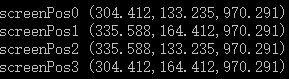和最终显示的结果一致。 完整的代码	//Perspective matrix test
/*
camera = new Camera(Vector3(0, 1, -10), 60, 16.0f / 9, 0.3f, 1000);
Matrix4x4 model = Matrix4x4::identity;

qDebug() <<"ProjectionMatri: " << camera->GetProjectionMatrix();
qDebug() << "ViewMatrix: " << camera->GetViewMatrix();
qDebug() << "ModelMatrix: " << model;

Vector3 vertice0(-0.5f, -0.5F, 0);
Vector3 vertice1(0.5f, 0.5F, 0);
Vector3 vertice2(0.5f, -0.5F, 0);
Vector3 vertice3(-0.5f, 0.5F, 0);

Matrix4x4 mvp = camera->GetProjectionMatrix() * camera->GetViewMatrix() * model;
//Vector3 tmp = mvp * vertice0;

Vector4 clipPos0 = mvp * Vector4(vertice0.x, vertice0.y, vertice0.z, 1);
Vector4 clipPos1 = mvp * Vector4(vertice1.x, vertice1.y, vertice1.z, 1);
Vector4 clipPos2 = mvp * Vector4(vertice2.x, vertice2.y, vertice2.z, 1);
Vector4 clipPos3 = mvp * Vector4(vertice3.x, vertice3.y, vertice3.z, 1);

qDebug() << "clipPos0" << clipPos0;
qDebug() << "clipPos1" << clipPos1;
qDebug() << "clipPos2" << clipPos2;
qDebug() << "clipPos3" << clipPos3;

Vector3 ndcPos0 = Vector3(clipPos0.x / clipPos0.w, clipPos0.y / clipPos0.w, clipPos0.z / clipPos0.w);
Vector3 ndcPos1 = Vector3(clipPos1.x / clipPos1.w, clipPos1.y / clipPos1.w, clipPos1.z / clipPos1.w);
Vector3 ndcPos2 = Vector3(clipPos2.x / clipPos2.w, clipPos2.y / clipPos2.w, clipPos2.z / clipPos2.w);
Vector3 ndcPos3 = Vector3(clipPos3.x / clipPos3.w, clipPos3.y / clipPos3.w, clipPos3.z / clipPos3.w);

qDebug() << "ndcPos0" << ndcPos0;
qDebug() << "ndcPos1" << ndcPos1;
qDebug() << "ndcPos2" << ndcPos2;
qDebug() << "ndcPos3" << ndcPos3;

int x = 0, y = 0, w = 640, h = 360, n = 0.3, f =1000;
Vector3 screenPos0(w * 0.5f * ndcPos0.x + x + w * 0.5f, h* 0.5f * ndcPos0.y + y + h *0.5f, 0.5f *(f - n) * ndcPos0.z + 0.5f * (f + n));
Vector3 screenPos1(w * 0.5f * ndcPos1.x + x + w * 0.5f, h* 0.5f * ndcPos1.y + y + h *0.5f, 0.5f *(f - n) * ndcPos1.z + 0.5f * (f + n));
Vector3 screenPos2(w * 0.5f * ndcPos2.x + x + w * 0.5f, h* 0.5f * ndcPos2.y + y + h *0.5f, 0.5f *(f - n) * ndcPos2.z + 0.5f * (f + n));
Vector3 screenPos3(w * 0.5f * ndcPos3.x + x + w * 0.5f, h* 0.5f * ndcPos3.y + y + h *0.5f, 0.5f *(f - n) * ndcPos3.z + 0.5f * (f + n));

qDebug() << "screenPos0" << screenPos0;
qDebug() << "screenPos1" << screenPos1;
qDebug() << "screenPos2" << screenPos2;
qDebug() << "screenPos3" << screenPos3;*/
正交投影在正交相机中，除了projection矩阵计算和透视相机的计算不一样，其他流程都一样。这里给出正交矩阵的计算代码.先看Unity，相机参数设置如下注意一下size指的是This is half of the vertical size of theviewing volume. Horizontal viewing size varies depending on viewport's aspectratio. 正交相机常常用于UI显示。对于一个640*360的屏幕，size就等于h/2 = 180.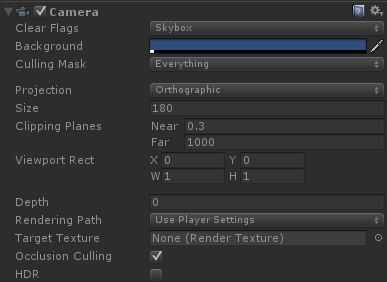这时候得到的透视矩阵是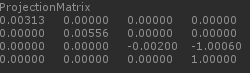C++代码Matrix4x4 Transform::OrthoFrustum(float l, float r, float b, float t, float zNear, float zFar)
{
Matrix4x4 result(0.0f);
result = 2 / (r - l);
result = 2 / (t - b);
result = -2 / (zFar - zNear);
result = 1;

//result = -(r + l) / (r - l);
//result = -(t + b) / (t - b);
result = -(zFar + zNear) / (zFar - zNear);

return result;
}
Matrix4x4 projection = Transform::OrthoFrustum(0.0f, static_cast<GLfloat>(creationFlags.width), 0.0f, static_cast<GLfloat>(creationFlags.height), 0.3, 1000);
qDebug() << "Orthographic ProjectionMatri: " << projection; 运行结果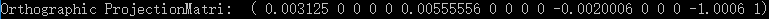后面计算的流程基本和透视投影的计算一致。至此，MVP完全搞定！链接详解MVP矩阵之齐次坐标和ModelMatrix详解MVP矩阵之齐次坐标和ViewMatrix详解MVP矩阵之齐次坐标和ProjectionMatrix 
展开全文游戏开发 算法
• 内置矩阵 支持的矩阵（float4x4）： ...UNITY_MATRIX_MVP 当前模型视图投影矩阵 UNITY_MATRIX_MV 当前模型视图矩阵 UNITY_MATRIX_V 当前视图矩阵UNITY_MATRIX_P 目前的投影矩阵 UNITY_MATRIX_VP 当前
• 基本信息mul函数mul函数，是表示矩阵M和向量V进行点乘，得到一个向量Z，这个向量Z就是对向量V进行矩阵变换后得到的值。 HLSL的mul函数接受mul(V, M)或mul(M, V),要注意通常HLSL要依DirectX计算(V * M)使用mul(V, M)...unity3d shader
• 【前言】 图形化调试可以加速开发。 例如在战斗中，可能需要知道所有单位的仇恨值，如果这些信息全打log的话，很难有...Unity中图形化调试主要4种 Debug.Draw Gizmos.Draw Graphic.DrawMesh GL 只需在Scene窗口显示的unity3d
• UNITY_MATRIX_MVP 当前模型视图投影矩阵 UNITY_MATRIX_MV 当前模型视图矩阵 UNITY_MATRIX_V 当前视图矩阵 UNITY_MATRIX_P 目前的投影矩阵 UNITY_MATRIX_VP 当前视图*投影矩阵 UNITY_MATRIX_T_MV 移调模型...shader unity3dunity3d 渲染管线3d引擎 Shader unity3d
• Unity-Shader-动态阴影(上) 投影的矩阵变换过程 【旧博客转移 - 2017年1月20日 01:20 】 前面的话 最近很长时间没写博文了，一是太忙 ( lan ) 了，二是这段时间又领悟了一些东西，脑子里很混乱...
• mul(UNITY_MATRIX_MVP, vector)" with "UnityObjectToClipPos(vector)" (vector can be an arbitrary expression)...unity Shader Unity5Unity3d 可编程渲染管线unity 游戏开发unity3d Shader